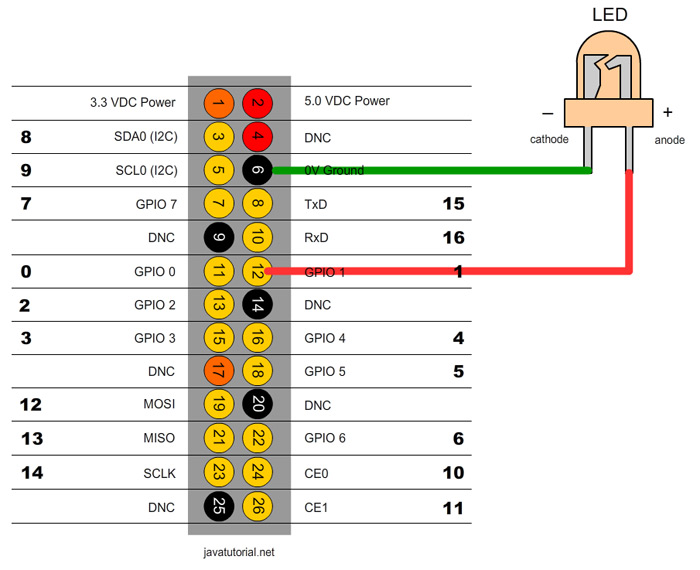Raspberry Pi Dim LED with PWM and Java

In this tutorial I will show you how to control the voltage using PWM in order to dim a LED.

Switching a LED on and off is easy. You can find out how to do this in my previous tutorial. Now I will show you how to control the level of voltage to make the LED light weaker or stronger. Normally Raspberry GPIOs use a voltage of 3.3. When the pin is up the output voltage is equal to 3.3 and when the pin is down the voltage is 0. So how to change the voltage to lets say 50% of 3.3V? We can do this using Pulse-width modulation (PWM). According to Wikipedia PWM is: “a technique used to encode a message into a pulsing signal. Although this modulation technique can be used to encode information for transmission, its main use is to allow the control of the power supplied to electrical devices, especially to inertial loads such as motors”.

In Raspberry Pi there is only one pin which supports hardware PWM. The hardware PWN pin produces a very clean signal. Although you can make a software PWM and all your pins to produce PWM. The software PWM pins don’t have such a clear signal and you have to make your own timings.

We will use exactly the same circuit as in the previous tutorial. Look at the diagram belowThe following code example will fade the LED to fully ON and than to fully OFF 3 times. I use the Pi4j API for the Java binding. If you want to know how to install, configure and run projects with Pi4j please refer to my previous tutorial.

import com.pi4j.wiringpi.Gpio;
import com.pi4j.wiringpi.SoftPwm;

public class DimLEDPWM {

private static int PIN_NUMBER = 1;

public static void main(String[] args) throws InterruptedException {
// initialize wiringPi library, this is needed for PWM
Gpio.wiringPiSetup();

// softPwmCreate(int pin, int value, int range)
// the range is set like (min=0 ; max=100)
SoftPwm.softPwmCreate(PIN_NUMBER, 0, 100);

int counter = 0;
while (counter < 3) {

// fade LED to fully ON
for (int i = 0; i <= 100; i++) {
// softPwmWrite(int pin, int value)
// This updates the PWM value on the given pin. The value is
// checked to be in-range and pins
// that haven't previously been initialized via softPwmCreate
// will be silently ignored.
SoftPwm.softPwmWrite(PIN_NUMBER, i);
}

// fade LED to fully OFF
for (int i = 100; i >= 0; i--) {
SoftPwm.softPwmWrite(PIN_NUMBER, i);
}

counter++;
}
}
}

You can run the program on Raspberry with following command:

sudo java -classpath .:classes:/opt/pi4j/lib/'*' DimLEDPWM